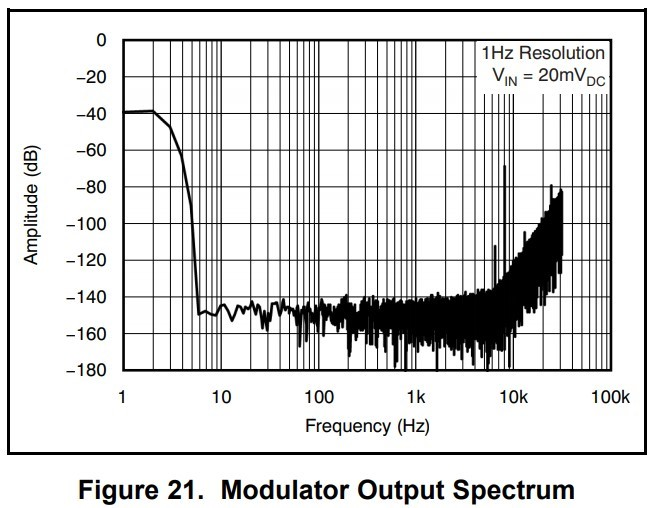If you have a related question, please click the "Ask a related question" button in the top right corner. The newly created question will be automatically linked to this question.

Hello，

Our customer need to find the PCM encode protocol files,  in addition, is the Y(n) of Equation 1 on datasheet Page 11 compatible with  PCM encode protocol?Best regards

Kailyn

• Hi Kailyn,

Unfortunately our expert on the ADS1281 has limited access to the internet at this time.  I'm not sure what you are asking for with respect to PCM encode protocol files.  This would seem to me more relevant for an audio codec as opposed to the ADS1281.  Can you give me more detail as to the customer application.  You can email me the details at:

pa_deltasigma_apps@ti.com

Best regards,

Bob B

• Hi Bob,

Greeting!
Pls check the file of  page 11, equation 1.
We set the PINMODE to "1" and make the ads1281 to ouput MO/M1 directly.
As the paper says, M0 is the PCM encode and the M1 is used to cancel the quantization noise.
Then , the Yn is generated from M0 and M1.
The questions is:
1: The theory or the circuit of M1 and Yn ?
2: Is Yn based on pcm encode? Yn is a bit?
3.What does "Yn" mean?
Thanks!
Best Regards！
• Hi Kailyn,

The M0 and M1 signals are serial bit streams that can be read directly at the output of the Delta-Sigma modulator, bypassing the built-in digital filter in case you wanted to implement your own digital filter (using a DSP or FPGA for example) with a different type of response.

Y[n] is an array of signed integers, representing the modulator's quantization level, where "n" represents the array index.

M0[n] and M1[n] are bit-streams (which can be represented as an array of 1's and 0's) and are combined according to equation 1 to calculate the quantization level. For example, the "6M0[n-3]" term is taking the the value of M0 from three samples ago and multiplying it by six. Note because of the "M0[n-4]" term, you have to collect at least four bits from both the bitstreams before you can calculate the integer value of "Y[n]".

• Hell Chris,

We have bypassed the built-in digital filter and use FPGA to  implement our own digital filter.

But we dont know the meaning of Yn and do not know how to use this as the input digital filter.

Could you explain this in detailed?

• Hi user6310626,

NOTES:

• To simply the conversion from the bit streams to voltages, I used "-1 in place of "0" values on M0 and M1. (NOTE: This is random data, since I didn't have a real dataset to use as an example).

• The M0 and M1 bits are summed together according to equation 1. Using "-1" in place of zeros, the integer values of Y can take on values between -49 and +49. (If you don't make this substitution, you'll get values between -24 and +25, which results in a small offset near mid-scale).

• The integer values for Y would then be passed into your digital filter and the output would need to be normalized to account for the filter gain. For this example, I just computed the average value of Y for the entire dataset...You should end up with a value between +/- 0.8.

• Next, I multiplied the average value of Y by a gain factor of 1.25 V/V since the modulator one's density only ranges from 10% to 90% (i.e. only 80% of the modulator output range is utilized) this compensates for the modulator's attenuation of the input signal.

• Finally, multiply that value by the full-scale voltage (+Vref / 2) and you have the average input voltage.

I hope that helps!

• Hello Chris,

Greeting!

I have tested it follow your instruction.However, there is something wrong with it.

As you know, 'Y' can take on values between -49 and +49.

For example, the input voltage is 1v and the ref is 5v.  We get the average value of Y per 2000 mclk period.

The We get the result is 610 or 646 etc.   The 1st voltage is (610/2000)*1.25*2.5=0.953125; The 2nd voltage is (646/2000)*1.25*2.5=1.009375‬;

The result is very different every time.

Could you tell us the reason and how long result will be stable?

In my opinion, the big change of  value of 'Y'  is the key.

Best Regards!

• Hi user6310626,

If I understand correctly, you've collected multiple data sets and in one case you calculated an average value of Y as 0.305 (= 610/2000) and another resulted in an average value of 0.323 (= 645/2000), is that correct?

From that description I don't see any unexpected behavior; however, I also don't know how much noise to expect when collecting the modulator data apart from what the noise spectrum looks like:Would you be able to share the data that you've collected? I think taking an FFT of the Y values and comparing it to Figure 21 (from the datasheet) would be helpful to see if you are measuring the expected amount of noise.

Also if you have a schematic of your circuit that you can share, that would also be very helpful to me! Feel free to send it to our email list: pa_deltasigma_apps@ti.com